# CBSE Sample Papers for Class 12 Maths UnSolved 2016 Set 6

0
1200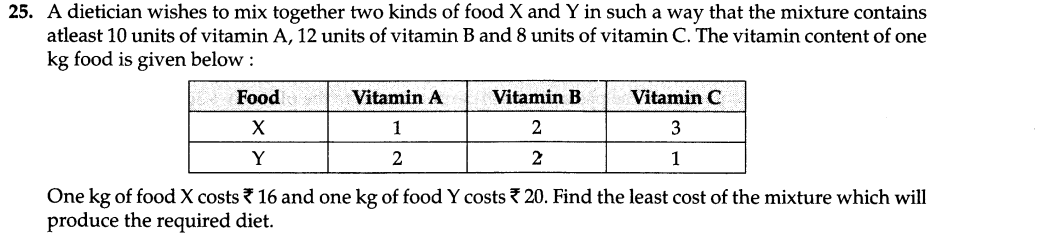SECTION —A

Question numbers 1 to 6 carry 1 mark each.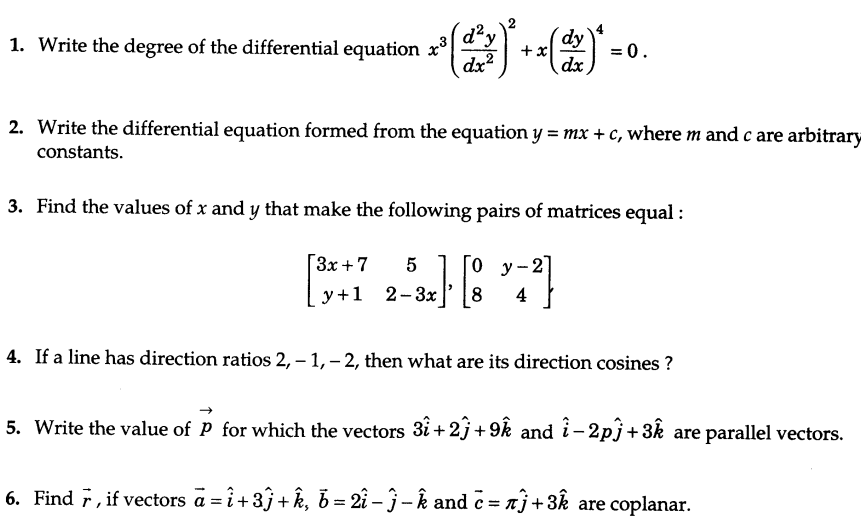SECTION —B

Question numbers 7 to 19 carry 4 mark each.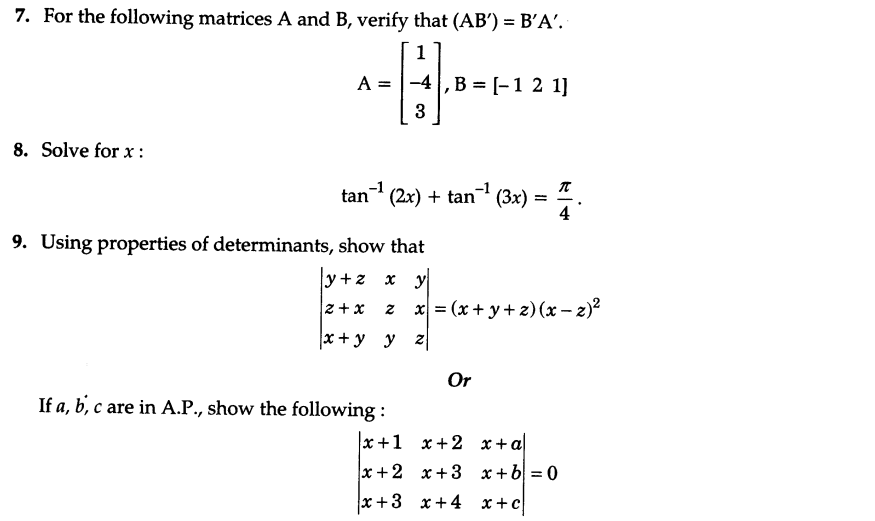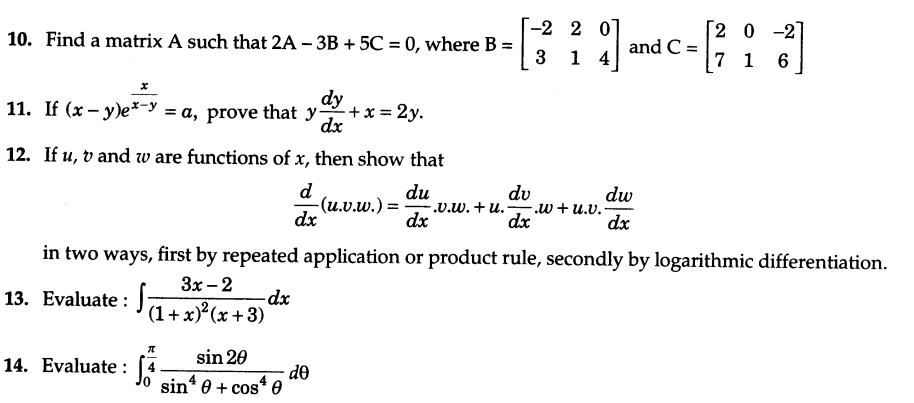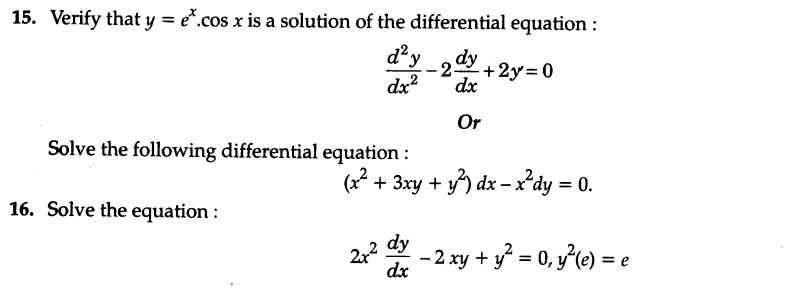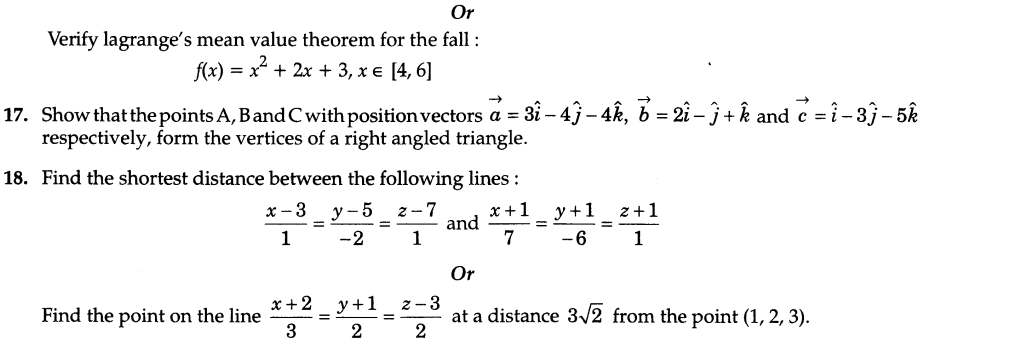19.A man is known to speak the truth 3 out of 4 times. He throws a dice and reports that it is a six. Find  the probability that it is actually a six.

SECTION — C

Question numbers 20 to 26 carry 6 marks each.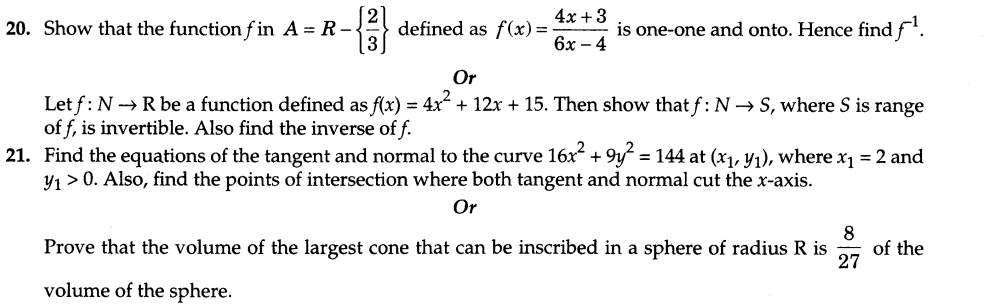22.Using the method of integration, find the area of the region bounded by the lines 2x + y = 4, 3x – 2y = 6, x – 3y + 5 = 0.
23.Find the point P on the curve y 2 = 4ax which is nearest to the point (11a, 0).
24.Show that the planes 2x+ y + 3z-2 = 0 and x – 2y + 5 = 0 are perpendicular to each other.26.An urn contains 25 balls of which 10 bear a mark ‘X’ and the remaining 15 bear a mark ‘Y’. A ball is drawn at random from the urn, its mark noted down and it is replaced. If 6 balls are drawn in this way, find the probability that
i)all will bear mark ‘X’,
ii)not more than two will bear ‘Y’ mark,
iii)at least one ball will bear ‘X’ mark and ‘Y’ mark will be equal.